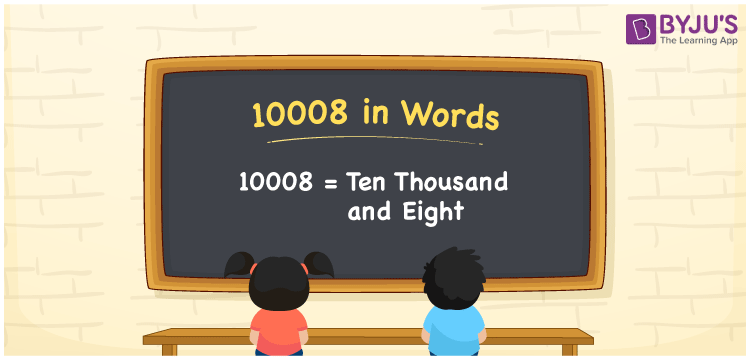# 10008 in Words

The word form of the number 10008 is nothing but the number name for 10008. Suppose you are asked to read the numeral 10008, written on a paper, then you may read it as “Ten thousand and eight”. Thus, we can write 10008 in words as Ten thousand and eight. Likewise, we can write the number names for any given cardinal number.

 10008 in words Ten thousand and eight Ten thousand and eight in Numbers 10008

## 10008 in English Words

Generally, we express numbers in words using the English alphabet. So, we spell 10008 in English words as “Ten thousand and eight”.## How to Write 10008 in Words?

The below table shows the place values for all the four digits of the number 10008.

 Ten thousands Thousands Hundreds Tens Ones 1 0 0 0 8

Here, ones = 8, tens = 0, hundreds = 0, thousands = 0, ten thousands = 1

The above digits can be written based on their place values as:

1 × Ten thousand + 0 × Thousand + 0 × Hundred + 0 × Ten + 8 × One

= 1 × 10000 + 0 × 1000 + 0 × 100 + 0 × 10 + 8 × 1

= 10000 + 8

= Ten Thousand + Eight

= Ten thousand and eight

10008 is a natural number that is the successor of 10007 and predecessor of 10009.

10008 in words – Ten thousand and eight

Is 10008 an odd number? – No

Is 10008 an even number? – Yes

Is 10008 a perfect square number? – No

Is 10008 a perfect cube number? – No

Is 10008 a prime number? – No

Is 10008 a composite number? – Yes

## Frequently Asked Questions on 10008 in Words

Q1

### How do you write 10008 in words?

We can write the number 10008 in words as “Ten thousand and eight”.
Q2

### How to write Rs. 10008 in words on a cheque?

On a cheque, an amount of Rs. 10008 can be written in words as “Ten thousand and eight rupees”.
Q3

### What number is Ten thousand and eight in words?

As we know, the number 10008 is Ten thousand and eight in word form.